Practice the AP 7th Class Science Bits with Answers Chapter 9 Reflection of Light on a regular basis so that you can attempt exams with utmost confidence.

## AP State Syllabus 7th Class Science Bits 9th Lesson Reflection of Light with Answers

Question 1.
Image of an object in a plane mirror is
A) red
B) inverted
C) small
D) virtual
D) virtual

Question 2.
Ray of light falling on the mirror is
A) reflected ray
B) refracted ray
C) deviated ray
D) incident ray
D) incident rayQuestion 3.
Angle of incidence
A) Angle of reflection
B) Angle of refraction
C) Angle of deviation
D) All the above
A) Angle of reflection

Question 4.
Distances of the object and image are ……….. in a plane mirror.
A) unequal
B) equal
C) may be equal or unequal
D) none of the above
B) equal

Question 5.
Army people use this
A) Kaleidoscope
B) Spectroscope
C) Periscope
D) All the above
C) Periscope

Question 6.
This is observed in a plane mirror
A) inverted image
B) small image
C) lateral inversion
D) All the above
C) lateral inversionQuestion 7.
Number of images formed when the object is placed in between the mirrors arranged at an angle of 90°
A) 3
B) 4
C) 5
D) 6
A) 3

Question 8.
Mirror used as a rearview mirror
A) Concave
B) Spherical
C) Plane
D) Convex
D) Convex

Question 9.
Mirror used by Dentists
A) Convex
B) Concave
C) Plane
D) Spherical
B) Concave

Question 10.
Mirror behind the bulb in a car headlight
A) Concave
B) Convex
C) Plane
D) None
A) ConcaveQuestion 11.
The ray of light falling on the mirror is called the
A) Incident ray
B) Reflected ray
C) Refracted ray
D) All of these
A) Incident ray

Question 12.
The ray of light returning from the mirror is called
A) Incident ray
B) Reflected ray
C) Refracted ray
D) All of these
B) Reflected ray

Question 13.
The angle between the normal and the reflected ray is called
A) Angle of reflection
B) Angle of incidence
C) Angle of refraction
D) Angle of circulation
A) Angle of reflection

Question 14.
The angle between the normal and the incident ray is called
A) Angle of reflection
B) Angle of incidence
C) Angle of refraction
D) Angle of circulation
B) Angle of incidenceQuestion 15.
Relationship between angle of incidence and the angle of reflection is
A) Equal
B) Not equal
C) Directly proportionate
D) Indirectly proportionate
A) Equal

Question 16.
If the angle of incidence is 20° with the normal, what will be the angle of reflection?
A) 20°
B) 40°
C) 70°
D) 90°
A) 20°

Question 17.
The mirrors in periscope are kept ………. to each other.
A) Horizontal
B) Vertical
C) Parallel
D) Linear
C) Parallel

Question 18.
In a mirror right of an object appears
A) Reverse
B) Right
C) Top
D) Left
D) LeftQuestion 19.
Number of mirrors required to provide multi images
A) 3
B) 5
C) 2
D) 6
C) 2

Question 20.
Number of images formed by the arrangement of plane mirrors with the angle of 60
A) 6
B) 7
C) 8
D) 5
D) 5

Question 21.
A) Concave mirror
B) Convex
C) Plane
D) Any one of these
A) Concave mirror

Question 22.
Dentist usually use ………… mirror.
A) Concave
B) Convex
C) Plane
D) All of these
B) ConvexQuestion 23.
Image on curved shining surface of inner portion is
A) Straight image
B) Mirror image
C) Reverse image
D) Irregular image
C) Reverse image

Question 24.
Outer portion of spoon acts as a ………… mirror.
A) Concave
B) Convex
C) Plane
D) Irregular
B) Convex

Question 25.
Clear images formed by ……………. reflection
A) Irregular
B) Diffused
C) Reverse
D) Regular
D) Regular

Question 26.
The number of images increased by the ………… of angle between two plane mirrors.
A) Increase
B) Reduction
C) Increase or decrease
D) None of these
B) ReductionQuestion 27.
In a ………… mirror we get diminished image of the object.
A) Concave
B) Plane
C) Rough
D) Convex
D) Convex

Question 28.
The image that can be obtained on a screen is called a
A) Virtual image
B) Reversed image
C) Enlarged image
D) Real image
D) Real image

Question 29.
Reflection from a smooth surface is called reflection
A) Irregular
B) Reverse
C) Regular
D) Irrespective
C) Regular

Question 30.
The image that can be viewed only in the mirror called
A) Virtual image
B) Reversed image
C) Enlarged image
D) Real image
A) Virtual imageQuestion 31.
What do we prove through this experiment?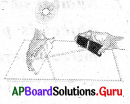A) Light rays
B) Light reflection
C) Pin hole camera
D) Lateral invertion
B) Light reflection

Question 32.
What is the source of light in this experiment?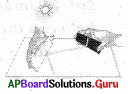A) Sun
B) Plain mirror
C) Mirror strip with the slit
D) Torch light
A) Sun

Question 33.
What is the instrument in that girl’s hand?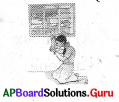A) Kaleidoscope
B) Periscope
C) Convex mirror
D) Concave mirror
B) PeriscopeQuestion 34.
What is the angle in between the two mirrors?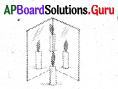A) 30°
B) 45°
C) 60°
D) 90°
D) 90°

Question 35.
The picture tells us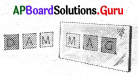A) Light reflection
B) Lateral invertion
C) Irregular reflection
B) Lateral invertion

Question 36.
How many plain mirrors do we need to prepare a periscope
A) 1
B) 2
C) 3
D) 4
B) 2Question 37.
What is the instrument in that boy’s hand?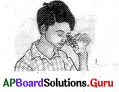A) Pin hole camera
B) Periscope
C) Kaleidoscope
D) Convex mirror
C) Kaleidoscope

Question 38.
What is the mirror used by this doctor?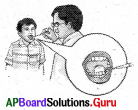A) Convex mirror
B) Concave mirror
C) Plain mirror
D) None of these
B) Concave mirror

Question 39.
If you want to farm multipe images how many mirrors atleastyou need?
A) 1
B) 2
C) 3
D) 4
B) 2Question 40.
When we reduce the angle between two mirrors the number of images
A) decrease
B) increase
C) does not change
D) none of these
B) increase

Question 41.
The image that can be obtained on a screen is called
A) real image
B) virtual image
C) multiple images
D) none of these
A) real image

Question 42.
Reflection from a rough surface is called
A) Real image
B) Virtual image
C) Regular reflection
D) Irregular reflection
D) Irregular reflection

Question 43.
The ray of light returning from the mirror is called
A) Incident ray
B) Reflected ray
C) Refracted ray
D) All of these
B) Reflected rayQuestion 44.
In which mirror we get diminished image of the object?
A) Concave
B) Plane
C) Rough
D) Convex
D) Convex

Question 45.
In this experiment the angle between the two plane mirrors is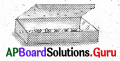A) 0°
B) 90°
C) 180°
D) 360°
A) 0°

Question 46.
Ravi observed the word FLOWER in the mirror. It appears like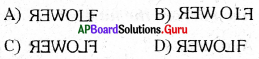C)Question 47.
Kavitha arranged the mirrors with 600 angle and got 5 images. Then Bhavana adjusted the mirrors and got 11 images. What is the angle between the mirrors?
A) 30°
B) 45°
C) 60°
D) 90°